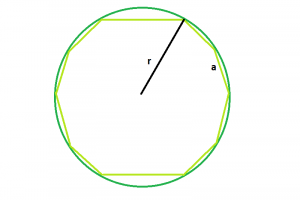# Area of decagon inscribed within the circle

Given here is a regular decagon, inscribed within a circle of radius r, the task is to find the area of the decagon.
Examples:

`Input: r = 5Output: 160.144Input: r = 8Output: 409.969`Approach
We know, side of the decagon within the circle, a = r√(2-2cos36)(Refer here
So, area of the decagon,

A = 5*a^2*(√5+2√5)/2 = 5 *(r√(2-2cos36))^2*(√5+2√5)/2=(5*r^2*(3-√5)*(√5+2√5))/4

Below is the implementation of the above approach:

## C++

 `// C++ Program to find the area of the decagon` `// inscribed within a circle` `#include ` `using` `namespace` `std;`   `// Function to find the area of the decagon` `float` `area(``float` `r)` `{`   `    ``// radius cannot be negative` `    ``if` `(r < 0)` `        ``return` `-1;`   `    ``// area of the decagon` `    ``float` `area = (5 * ``pow``(r, 2) * (3 - ``sqrt``(5))` `                  ``* (``sqrt``(5) + (2 * ``sqrt``(5))))` `                 ``/ 4;` `    ``return` `area;` `}`   `// Driver code` `int` `main()` `{` `    ``float` `r = 8;` `    ``cout << area(r) << endl;`   `    ``return` `0;` `}`

## Java

 `// Java Program to find the area of the decagon ` `// inscribed within a circle `   `import` `java.io.*;`   `class` `GFG {` `    `  `// Function to find the area of the decagon ` `static` `double` `area(``double`  `r) ` `{ `   `    ``// radius cannot be negative ` `    ``if` `(r < ``0``) ` `        ``return` `-``1``; `   `    ``// area of the decagon ` `    ``double`  `area = (``5` `* Math.pow(r, ``2``) * (``3` `- Math.sqrt(``5``)) ` `                ``* (Math.sqrt(``5``) + ((``2` `* Math.sqrt(``5``))))/ ``4``); ` `    ``return` `area; ` `} `   `// Driver code ` `    `  `    ``public` `static` `void` `main (String[] args) {` `        ``double`  `r = ``8``; ` `        ``System.out.println (area(r)); ` `    ``}` `//This code is contributed by ajit` `}`

## Python3

 `# Python3 Program to find the area of` `# the decagon inscribed within a circle` `from` `math ``import` `sqrt,``pow`   `# Function to find the ` `# area of the decagon` `def` `area(r):` `    `  `    ``# radius cannot be negative` `    ``if` `r < ``0``:` `        ``return` `-``1`   `    ``# area of the decagon` `    ``area ``=` `(``5` `*` `pow``(r, ``2``) ``*` `(``3` `-` `sqrt(``5``)) ``*` `                 ``(sqrt(``5``) ``+` `(``2` `*` `sqrt(``5``))))``/` `4` `    ``return` `area`   `# Driver code` `if` `__name__ ``=``=` `'__main__'``:` `    ``r ``=` `8` `    ``print``(area(r))`   `# This code is contributed` `# by Surendra_Gangwar`

## C#

 `// C# Program to find the area of the ` `// decagon inscribed within a circle ` `using` `System;`   `class` `GFG` `{` `        `  `// Function to find the area ` `// of the decagon ` `static` `double` `area(``double` `r) ` `{ `   `    ``// radius cannot be negative ` `    ``if` `(r < 0) ` `        ``return` `-1; `   `    ``// area of the decagon ` `    ``double` `area = (5 * Math.Pow(r, 2) * ` `                  ``(3 - Math.Sqrt(5)) * ` `                      ``(Math.Sqrt(5) + ` `                 ``((2 * Math.Sqrt(5))))/ 4); ` `    ``return` `area; ` `} `   `// Driver code ` `static` `public` `void` `Main ()` `{` `    ``double` `r = 8; ` `    ``Console.WriteLine (area(r)); ` `}` `}`   `// This code is contributed by akt_mit`

## Javascript

 ``

## PHP

 ``

Output

```409.969
```

Time complexity: O(1)

Auxiliary Space: O(1), since no extra space has been taken.

Feeling lost in the world of random DSA topics, wasting time without progress? It's time for a change! Join our DSA course, where we'll guide you on an exciting journey to master DSA efficiently and on schedule.
Ready to dive in? Explore our Free Demo Content and join our DSA course, trusted by over 100,000 geeks!

Previous
Next